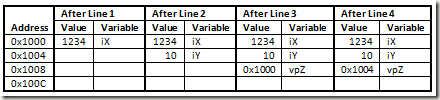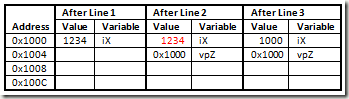## What is ‘void’?

I have often seen that there is a confusion around the ‘void’ concept in C++ among the new comers to C++. Especially when it is used as a pointer. A commonly seen definition is that void means lack of something. Hence you cannot have a variable of type void. But you can have a pointer which is of void type. This is a bit confusing, so I am going to try and give examples and explain about each one.

Before I start, a pointer stores the address to some content. So, the size of all pointers are the same regardless of the content they point to. However, the pointer arithmetic depends on the type of content it is pointing to. Here I am going to talk about a type that means nothing and can be used to point to any type.

#### 1. void vX;

This is NOT allowed in C++. You cannot have a variable of type void. This makes some sense, void means nothing, hence you cannot declare a variable of type nothing. A variable is a name for a location in memory, and since void does not define the amount of memory needed you cannot allocate it.

#### 2. void fnDoSomething(void);

This is allowed in C++. This says that the function fnDoSomething accepts nothing and returns nothing. There is no memory  needed to store the variables here. However, void fnDoSomething(int iX, void); is invalid. This is trying to say fnDoSomething accepts an integer and the next argument is nothing. The compiler will show an error indicating that the type of the second argument is not known or invalid use of void. Since we are passing values the compiler has to put code in there to copy the value that will go into ‘iX’, so before copying memory has to be allocated for that argument. The first argument is fine because the compiler knows the cost of int and it can allocate the amount of memory needed for it. When the compiler looks at the second argument, it cannot allocate memory because of the problem we saw in section 1. Finally, void fnDoSomething(); has the same meaning as void fnDoSomething(void); . Here is a simple example:

`   1:  void fnSomething(void)`
`   2:  {`
`   3:      cout << "Something was done.";`
`   4:  }`

#### 3. void *vpX;

This is allowed in C++. First, a pointer is being declared. This is allowed but NOTvoid vX;’ because here a pointer was created and we are telling the compiler not to care about the type of the item it is pointing to. Usually, the amount of memory for any type of pointer is 4 bytes (8 bytes for x64). Second, since it is a pointer the compiler knows the amount of memory it needs to allocate, and ‘vpX’ is the name for that allocated memory.  A void pointer can point to any variable (except for those which are const or volatile) or can point to a function (except for member functions of a class).  Here is an example, the program  prints the byte sequence from memory for int and double data types using the same function.

`   1:  void fnPrintHex(void *vpData,int iSize)`
`   2:  {`
`   3:      unsigned char* cpData = (unsigned char*)vpData;`
`   4:      for(int i=iSize-1;i>=0;i--)`
`   5:          cout << hex << (int)*(cpData+i);`
`   6:  }`
`   7:   `
`   8:  int main()`
`   9:  {`
`  10:      int iX =1234;`
`  11:      fnPrintHex(&iX,sizeof(iX));`
`  12:      cout << endl;`
`  13:   `
`  14:      double dX = 1234.5678;`
`  15:      fnPrintHex(&dX,sizeof(dX));`
`  16:      cout << endl;`
`  17:   `
`  18:      return 0;`
`  19:  }`

#### 4. const void *vpX;

This is allowed in C++. This means that the void pointer points to a constant value. The following will cause a type-cast error during compilation:

`   1:  const int iX = 10;`
`   2:  void *vpX = &iX; // Type cast error`

Only a const void type pointer is allowed to point to a constant. This means that the pointer itself is NOT constant and its value can be changed. But, the value it is pointing to is supposed to be constant. Note, const T *pX = &X is valid, T here is the same type as X. In the following code, in line 3 the ‘vpZ’ pointer is assigned the address of ‘iX’ and in line 4 it is reassigned with address of ‘iY’. The following code should print 1234 and 10, on separate lines.

`   1:  const int iX = 1234;`
`   2:  const int iY = 10;`
`   3:  const void *vpZ = &iX;`
`   4:  vpZ = &iY;`
`   5:  cout << iX << endl << *(const int*)vpZ;`There is a minor issue here. Note that in the above code both ‘iX’ and ‘iY’ are constants and their value cannot be changed. But, you’ll see that you can do something as shown in the code below. If you read the code it may seem like it’ll print 1000 and 1000 on separate lines. Well it actually prints 1234 and 1000 on separate lines. Constants are optimized, hence the value of ‘iX’ gets placed wherever it is used. But, the original memory location is still alterable since it was type-cast to a editable type. Note, some debuggers will show the value of ‘iX’ on line 4 as 1000, you may have to test this on your compiler and debugger.

`   1:  const int iX = 1234;`
`   2:  const void * vpZ = &iX;`
`   3:  *(int*)vpZ = 1000;`
`   4:  cout << iX << endl << *(int*)vpZ;`

####In the figure above, the number is highlighted in red because the compiler would have already picked up the value and applied everywhere ‘iX’ is used. So even if the value is changed it no longer matters wherever ‘iX’ used.

#### 5. void *const vpX;

This is allowed in C++. This means that the void pointer itself is a constant but it points to a variable. So, once an address is assigned to the pointer, you cannot reassign another address to it. In line 3 an address is assigned to ‘vpZ’ and after that line ‘vpZ’ cannot be assigned another address.

`   1:  int iX = 1234;`
`   2:  int iY = 10;`
`   3:  void *const vpZ = &iX;`
`   4:  vpZ = &iY; // This is NOT allowed`

But, the content at the address where the void pointer points to can be changed. You need to type-cast before you can make any changes. See the following code, it prints out 1000 and 1000, on separate lines.

`   1:  int iX = 1234;`
`   2:  void *const vpZ = &iX;`
`   3:  *(int*)vpZ = 1000;`
`   4:  cout << iX << endl << *(int*)vpZ;`

#### 6. const void* const vpX;

This is allowed in C++. This is a void pointer which is a constant pointing to an address whose contents are also constant. This is a combination of section 4 and 5 and the same rules apply. The following code will fail to compile, see section 5 for the reason:

`   1:  const int iX = 1234;`
`   2:  const int iY = 5432;`
`   3:  const void *const vpZ = &iX;`
`   4:  vpZ = &iY; // This is NOT allowed`

But the following code is allowed, see section 4 for the reason:

`   1:  const int iX = 1234;`
`   2:  const void *const vpZ = &iX;`
`   3:  *(int*)vpZ = 1000;`
`   4:  cout << iX << endl << *(int*)vpZ;`

I tested all of my code in visual C++ and GNU GCC. Please let me know if there are any mistakes. This is an introduction, I’ll try and write more advanced usage of void pointers in the future. Thanks for your patience.